# Team:Grenoble-Alpes/Temperature Control

Engineering

## Temperature acquisition

##### The first step was to find a way to detect temperature variations in order to adjust the temperature inside the kit. A specific type of resistance thermometer was used: the Pt100 sensor. In fact, its internal variable resistance changes linearly with the temperature variation. At 0°C, the value of Pt100’s resistance is equal to 100 Ω. But, the problem is that working with resistance variations is not that easy. This is why a continuous current source was added thanks to the LM334 component so as to work with voltage variations.##### The current passing through the Pt100 is set with the LM334, so the resistance variation is converted into a voltage variation. To choose that current, it is recommended to fix it under 1mA, so as to avoid burn it. This is why a resistance Rset was added, which value is given by the following equation.## Offset regulation

##### Now, even though the system was designed to have 0°C at 0V, it was not the case, an offset was observed. To compensate this offset, a voltage divider is needed. A reference tension is created, from which the tension related to the temperature is subtracted.##### At 0°C, the Pt100 resistance should be equal to 100 Ω and the current which passes through this component is 100µA. Thanks to the Ohm’s relation, the tension can be deduced and it must be equal to 10 mV. The following equation gives the relation between R1 , R2 and VR2.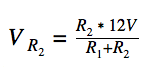##### Now, as explained before, the reference tension and the tension related to the actual temperature have to be subtracted. The difference between these two tensions is in the millivolts range which is a little bit too small to be used: the difference was therefore amplified with the AD8223 instrumentation amplifier. This device subtracts the two inputs and amplifies the result by a gain which is defined by the following relation.##### where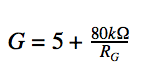##### As the Arduino card can’t record tensions higher than 5V, RG was chosen in order to have the output tension included between 0 and 5 V, to use as much as possible the band free tension. As the maximum tension coming from the Pt100 is 12,899 mV (which corresponds to a temperature of 75°C), the resistance fixing the gain can be deduced with the following relations.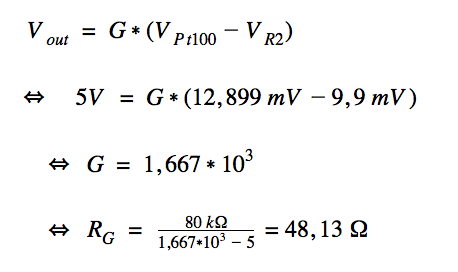##### Finally, to protect AD8223 from too high currents, a resistance was added. The Pt100 is a fragile element, so in case it would be disconnected/broke, this new resistance would receive the current from the LM334 instead of going through the AD8223 component and breaking it.## Temperature enslavement

##### A Peltier device is a component which turns current into temperature differences. It can heat or cool according to the sign of this current. Peltier device can be considered as a resistance, so we can consider the tension as the element determining whether the device should heat or cool. However, the output Arduino tension can only be positive, so a power converter needs to be used to make sure it remains positive. Accordingly, a power converter is needed to deliver positive or negative tensions with an input tension that is only positive, because as said before, the output Arduino tension can only be positive. L298 is a power converter and it is the component that we chose.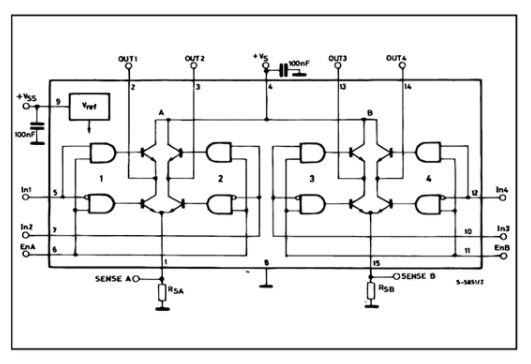##### Now, in the Arduino card, according to the sign of the difference between the two analogue tensions (the one related to the temperature within the kit and the other related to the wanted temperature), the hardware determines whether the Peltier device has to heat or cool the system. Therefore, it fixes the value of In1 and In2. According to the value of this difference, the hardware generates a PWM sent to EnA. The complete electronic circuit is showed below.##### A SMD card has been realised so further tests could be performed.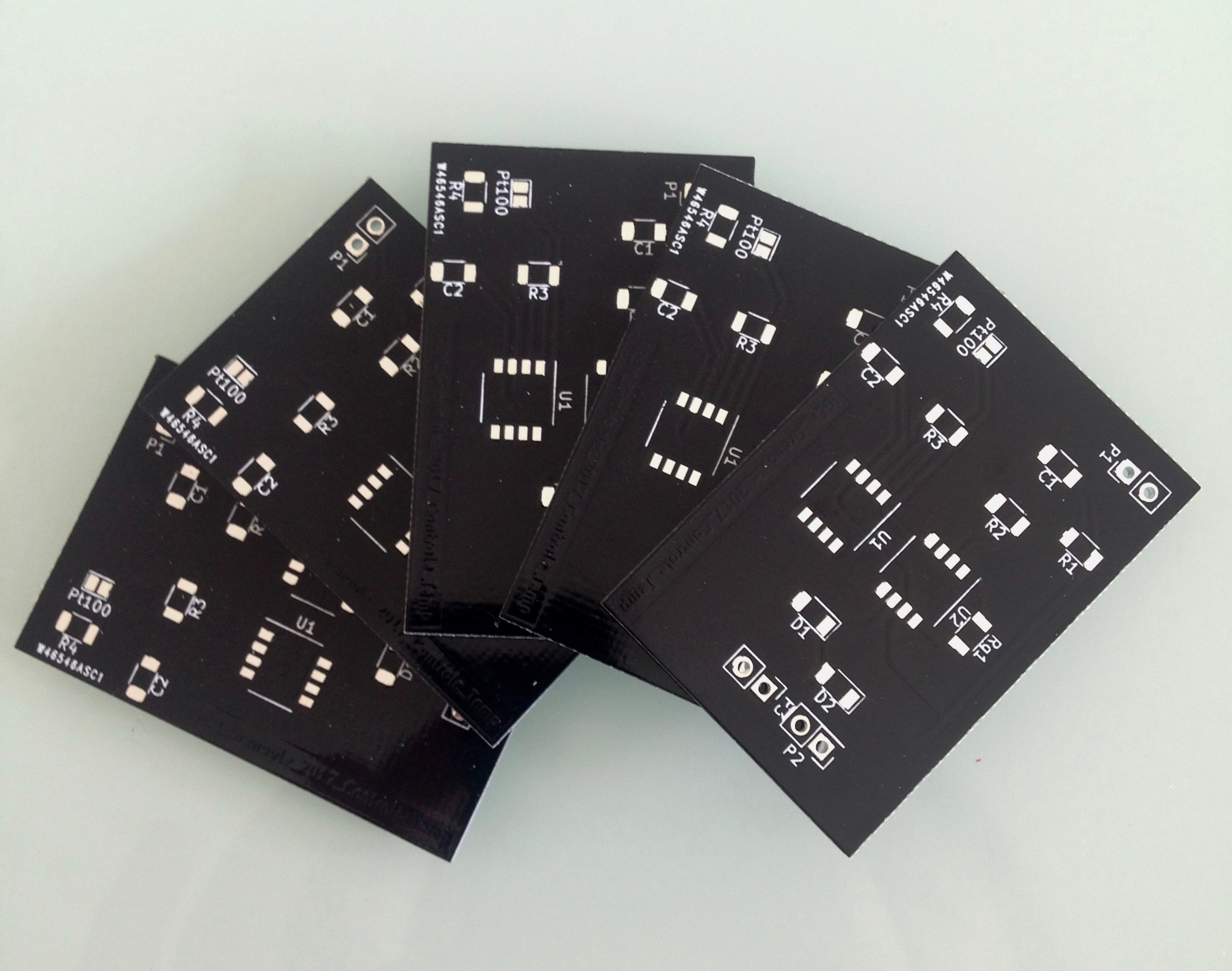## Results

##### To confirm our argumentation, we realised the circuit on an operating console and verified, step by step, that the tensions expected were satisfying. However, due to a lack of time, the SMD card, and so the temperature enslavement, was not tested. The next step would be to test this card, allowing to make further improvements if necessary.

Designed by iGEM Grenoble-Alpes 2017 team | Site Map